# What is the threshold frequency ν0 of cesium? Note that 1 eV (electron volt)=1.60×10−19 J. Express your answer numerically in hertz

What is the threshold frequency ν0 of cesium? Note that 1 eV (electron volt)=1.60×10−19 J.

Concepts and reason
Calculate the threshold frequency by using the following equation.Here, h is plank constant,is threshold frequency, and E is ionization energy.
Calculate the threshold frequency by using the values of plank constant and the energy.

Fundamentals
Minimum amount of frequency that causes photo electric emission is called as threshold frequency.
Emission of electrons when light is incident on surface of a material is called as photo emission and this effect is called as photo electric effect.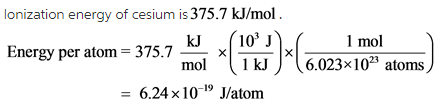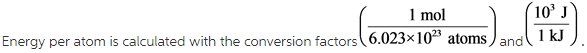Calculate the threshold frequency by substituting the values in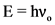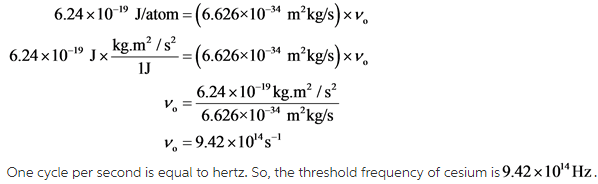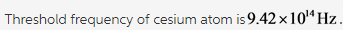The value of plank constant in the units of kilograms is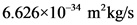. Substitutefor h and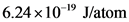for E in the formula. Value of threshold frequency is obtained in the units of inverse of second. One inverse of second is equal to on hertz.
Threshold frequency of cesium atom is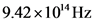.